# Preface

This chapter gives an introduction to recurrences and representing/approximating solutions to nonlinear and linear variable coefficient differential equations as power series. There are several methods to obtain a power series solution, and they all lead to some iteration schemes and solving recurrences. The iteration procedure is conceptually very basic, and sets the tone for a large class of numerical integration techniques. Also, when the problem is cast in this way, many of the properties of the solution can be investigated without explicitly solving the differential equation.

The majority of this chapter is devoted to determination of solutions in terms of convergent power series. The set of functions represented by power series, called the holomorphic functions, is denoted by 𝓗(𝑎,b) and it is a proper subset of C(𝑎,b) of infinitely differentiable functions on the interval (𝑎,b). The word derives from the Greek ο λ ο σ (holos), meaning "whole," and μ ο ρ φ η (morphe), meaning "form" or "appearance."

It should be noted that a technique for (analytically) calculating the power series of the solution to differential equations (in terms of the initial value parameters) is sometimes referred to as the differential transformation method (DTM). Such jargon is mostly appropriate for users who are involved in the elaboration of software packages---numerical routines---for automatically solving ordinary differential equations. The main ingredient of the Taylor method is a transformation of a differential equation into a difference equation that is iteratively solvable.

# Series and Recurrences

Owing to the complicated structure of some ordinary differential equations, it is not always possible to obtain the corresponding solution of an initial value problem in a reasonable form. In such situations, we need to resort to methods that produce an approximate solution, of which we choose the form of an infinite series. There are some reasons why we go in this way. First of all, historically speaking power series were the first tool to approximate functions, and this topic is part of the calculus course. Second, when a function is represented (or approximated) by a power series, all its coefficients are determined by derivatives evaluated at one point. So only infinitesimal knowledge of the function at one single point is needed to find all coefficients of the corresponding power series. This makes Taylor series an appropriate technique for solving initial value problems because solutions are also determined by the the initial conditions imposed at one single point. Since a power series converges within a symmetrical interval $$\left\vert x - x_0 \right\vert < R,$$ it is usually referred to as a local method. In what follows, we illustrate a procedure of this type, based on series expansions for functions of a real variable.

The topic of this chapter is roughly divided into three parts. Since applications of power series for solving differential equations lead to developing and solving recurrences for its coefficients, the first part is devoted to difference equations and generating functions. In the second part, we present three approaches to solve nonlinear differential equations: power series, Picard's iteration, and the Adomian decomposition method. The rest of the chapter is about solving linear differential equations with variable coefficients. The majority of it is devoted to series representations of solutions for equations with regular singular points, developed by the German mathematicians Lazarus Fuchs (1833--1902) in 1866 and Ferdinand Frobenius (1849--1917) in 1873.

Until now, we discussed methods for solving second and higher order constant coefficient differential equations. In applications, higher order nonlinear equations and linear equations with variable coefficients are just as important, if not more so, than equations with constant coefficients. In this chapter, we turn our attention to variable coefficient linear differential equations, and nonlinear equations. Out of these, the second order equations play a crucial role for several reasons. First of all, they are the most simple equations to analyze. Secondly, the second order equations are frequently used in applications, and thirdly, the majority of other equations can be reduced to these equations. For example, the Riccati equation is equivalent to a second order differential equation with variable coefficients. Moreover, the methods involved in solving differential equations of the second order can be easily extended to the higher order equations.

Once we done with nonlinear equations, we turn our attention to linear differential equations with variable coefficients. Here the Fuchs method will be our main tool. The procedure is similar to the use of undetermined coefficients for polynomial solutions, except that there are infinitely many coefficients. In practical applications, we don't need to determine all of them, but some finite number. Equating coefficients of like powers, we obtain the recursive relation for determination of values of coefficients in terms of its predecessors. Before computers were available, it was very tedious procedure that limited their applications. Now we can dedicate this job to a computer algebra system.

Taylor's Series

If a real-valued function f(x) has N+1 continuous derivatives on the interval 𝑎 ≤ xb, then

\begin{equation} f(x) = \sum_{n=0}^N c_n \left( x - x_0 \right)^n + R_{N+1} = \sum_{n=0}^N \frac{1}{n!}\, f^{(n)} \left( x_0 \right) \left( x - x_0 \right)^n + R_{N+1} (x), \qquad a < x < b, \label{EqTaylor.1} \end{equation}
where the coefficients cn are (linear) functionals on the space of holomorphic functions, cn : 𝓗 → ℝ, defined by
\begin{equation} \label{EqTaylor.2} c_n = c_n (f) = \frac{1}{n!}\,\lim_{x\to x_0} \,\texttt{D}^n f (x) = \frac{1}{n!}\,\lim_{x\to x_0} \,\frac{{\text d}^n f(x)}{{\text d} x^n} , \end{equation}
and the remainder term is
\begin{equation} R_{N+1} (x) = \frac{f^{(N+1)} (\xi )}{(N+1)!} \left( x - x_0 \right)^{N+1} = \frac{1}{N!} \int_{x_0}^x \left( x-t \right)^N f^{(N+1)} (t)\,{\text d}t \label{EqTaylor.3} \end{equation}
for some (unknown) ξ in the interval 𝑎 ≤ ξ ≤ xb. The latter form of reminder is often arrived at by a succession of integration by parts; the former remainder, called after Lagrange, is derived by an application of Rolle's theorem or the mean-value theorem to a suitable function. Note that ξ in the Lagrange reminder is not merely a constant, but it is a function of the endpoint x.

If we set RN+1 = 0, an N-th degree polynomial approximation to f(x) is obtained:

\begin{equation} \label{EqTaylor.7} T_N (x) = \sum_{n=0}^N c_n \left( x - x_0 \right)^n , \qquad c_n = \left[ x^n \right] f(x) = \frac{1}{n!}\,f^{(n)} \left( x_0 \right) , \quad n= 0,1,2,\ldots , N. \end{equation}
Here we use the standard notation $$\displaystyle \left[ x^n \right] f(x)$$ to extract n-th coefficient of the Taylor series expansion for function f(x). This polynomial TN(x) is called the Taylor polynomial of degree N for the function f(x). If the center x0 is chosen to be zero, the corresponding polynomial is usually referred to as the Maclaurin polynomial. The magnitude of RN+1 provides an error estimate for this polynomial which can be found if a suitable bound on $$\left\vert f^{(N+1)} (\xi ) \right\vert$$ over [𝑎,b] is known.

To develop Taylor's series for a smooth function f(x) centered at an initial point 𝑎, we begin with the identity
$\int_a^x f' (x) \,{\text d}x = f(x) - f(a) , \tag{M}$
where we use x for a variable and for a dummy character of integration. Solving for f(x), we obtain
$f(x) = f(a) + \int_a^x f' (x) \,{\text d}x .$
If we replace f(x) by its derivative f'(x), we get
$f'(x) = f'(a) + \int_a^x f'' (x) \,{\text d}x .$
Putting this expression for the derivative into the previous integral gives
\begin{align*} f(x) &= f(a) + \int_a^x \left[ f'(a) + \int_a^x f'' (x) \,{\text d}x \right] {\text d}x \\ &=f(a) + f' (a) \left( x - a \right) + \int_a^x {\text d}x \int_a^x {\text d}x \,f'' (x) . \end{align*}
Next, replacing f'(x) by its second derivative f''(x) in the main formula (M) leads to
$f''(x) = f''(a) + \int_a^x f''' (x) \,{\text d}x$
and putting this expression into the previous integral gives
\begin{align*} f(x) &=f(a) + f' (a) \left( x - a \right) + \int_a^x {\text d}x \int_a^x {\text d}x \,\left[ f''(a) + \int_a^x f''' (x) \,{\text d}x \right] \\ &= f' (a) \left( x - a \right) + \frac{f'' (a)}{2!} + \int_a^x {\text d}x\int_a^x {\text d}x \int_a^x {\text d}x \,f''' (x) . \end{align*}
Repeating this process (assuming that f is sufficiently differentiable on the given interval), we obtain
$f(x) = f(a) + f' (a) \left( x - a \right) + \frac{f'' (a)}{2!} + \cdots + \frac{f^{(n-1)} (a)}{(n-1)!} + R_n (x) ,$
where the remainder term is
$R_n (x) = \int_a^x {\text d}x \cdots \int_a^x {\text d}x \,f^{(n)} (x) .$
The n-dimensional integral in the right hand side can be reduced to the one-dimensional integral using integration by parts
$R_n (x) = \frac{1}{(n-1)!} \int_a^x {\text d}t \, \left( x-t \right)^{n-1} f^{(n)}(t) ,$
which gives an integral form of the remainder. We prove the above integral form for the remainder using mathematical induction. The basic term n = 1 was proved previously.

Now we suppose that the formula is true for n = k, that is,

$R_k (x) = \frac{1}{(k-1)!} \int_a^x \left( x-t \right)^{k-1} f^{(k)} (t) \,{\text d}t .$
We want to show that it’s true for n = k+1, that is,
$R_{k+1} (x) = \frac{1}{k!} \int_a^x \left( x-t \right)^k f^{(k+1)} (t) \,{\text d}t .$
Again we use integration by parts, this time with u = (x - t)k and $${\text d}v = f^{(k+1)} (t) \,{\text d}t .$$ Then $${\text d}u = -\left( k+1 \right) (x-t)^k$$ and $$v = f^{(k)} (t) ,$$ so
\begin{align*} \frac{1}{k!} \int_a^x \left( x-t \right)^k f^{(k+1)} (t) \,{\text d}t &= \left. \frac{1}{k!} \left( x-t \right)^k f^{(k+1)} (t) \right\vert_{t=a}^{t=x} + \frac{k}{k!} \int_a^x \left( x-t \right)^{-1}k f^{(k+1)} (t) \,{\text d}t \\ &= 0 - \frac{1}{k!} \left( x-a \right)^k f^{(k+1)} (a) + \frac{1}{(k-1)!}\int_a^x \left( x-t \right)^{k-1} f^{(k)} (t) \,{\text d}t \\ &= - \frac{1}{k!} \left( x-a \right)^k f^{(k+1)} (a) + R_k (x) \\ &= f(x) - T_k - \frac{1}{k!} \left( x-a \right)^k f^{(k+1)} (a) \\ &= f(x) - T_{k+1} (x) = R_{k+1} (x) . \end{align*}
Therefore, the integral form of the remainder is true for n = k+1 when it is true for n = k. Thus, by mathematical induction, it is true for all n.

To obtain an alternative form for the remainder Rn, suppose that $$f^{(n)} (x)$$ has a minimum value m and a maximum value M on the closed interval [𝑎,b]. Then,

$m \int_a^x {\text d}x \cdots \int_a^x {\text d}x \le R_n (x) \le M \int_a^x {\text d}x \cdots \int_a^x {\text d}x$
or, upon carrying the integration,
$\frac{m}{n!} \left( x - a \right)^n \le R_n (x) \le \frac{M}{n!} \left( x - a \right)^n .$
If we assume that $$f^{(n)} (x)$$ is continuous on [𝑎,x], then it can be shown that it must take on all values, from its minimum m to its maximum M, over the closed interval. It therefore follows that the remainder must be expressed as
$R_n (x) = \frac{f^{(n)} (\xi )}{n!} \left( x- a \right)^n$
for some suitable point ξ from [𝑎,x]. This gives the Lagrange form of the remainder. Joseph-Louis Lagrange obtained the remainder formula in a 1772 article published in Nouveaux Mémoires de l’Académie Royale des Sciences et Belles-Lettres de Berlin. It seems that Lagrange was the first to study the conditions to expand a function in Taylor series, published in his book Théorie des Fonctions Analytique, 1797.

The Taylor's series for a function having infinitely many derivatives is obtained by taking the limit N → ∞,
\begin{equation} f(x) = \sum_{n\ge 0} c_n \left( x - x_0 \right)^n = \sum_{n\ge 0} \frac{f^{(n)} \left( x_0 \right)}{n!} \left( x - x_0 \right)^n , \qquad -r < x - x_0 < r , \label{EqTaylor.4} \end{equation}
subject the remainder term tends to zero. This series for arctan x was formulated by the Scottish mathematician and astronomer James Gregory in his book Geometriae Pars Universalis (1668). In the same year, N. Mercator gave the series expansion of ln(1 +x) in his Logarithmotechnia and I. Newton obtained the series expansion for (1 +x)α, sin x, cos x and exp x, which appeared in the correspondence with Leibniz in 1676. What is less well-known is that the Indian Kerala school founded by Madhava of Sangamagrama have already knew the series expansion of these functions. It seems that the first mathematician to give a general formula for series expansion of a function was Johann Bernoulli in 1694 (published in Acta eruditorum). It was Joseph-Louis Lagrange who called this series after B. Taylor.

In 1715, the English mathematician Brook Taylor formally introduced the formula $$f(x+h) = f(x) + h\,f'(x) + \cdots + \frac{h^n}{n!}\,f^{(n)} (x) + \cdots ,$$ without any conditions for the validity of such representation. To obtain such formula, Taylor used the theory of finite differences. If the Taylor series is centered at zero, then that series is also called a Maclaurin series, after the Scottish mathematician Colin Maclaurin, who made extensive use of this special case of Taylor series in his Treatise of Fluxions of 1742. The necessary and sufficient conditions for this series to exist and to sum to the function f(x) is that RN+1 → 0 as N → ∞. The point x0 is usually called the center of Taylor's series. By changing the variables t = x - x0, any Taylor's series can be transferred to a Maclaurin series. The above power series representation is known as the local expansion because its terms are determined by infinitesimal behavior of the function f(x) at one single point---its center x0.

Example: The total relativistic energy of a particle of mass m and velocity v is

$E = mc^2 \left( 1 - \frac{v^2}{c^2} \right)^{-1/2} .$
We expand this function into the binomial series
$\left( 1 + x \right)^n = 1 + n\,x + \frac{n \left( n-1 \right)}{2!}\, x^2 + \frac{n \left( n-1 \right) \left( n-2 \right)}{3!}\, x^3 + \cdots ,$
with x = -v²/c² and n = -½, we get
\begin{align*} E &= m\,c^2 \left[ 1 - \frac{1}{2} \left( - \frac{v^2}{c^2} \right) + \frac{(-1/2)\,(-3/2)}{2!} \left( - \frac{v^2}{c^2} \right)^2 + \frac{(-1/2)\,(-3/2)\,(-5/2)}{3!} \left( - \frac{v^2}{c^2} \right)^3 + \cdots \right] , \end{align*}
or
$E = m\,c^2 + \frac{1}{2}\, m\,v^2 + \frac{3}{8}\, m\,v^2 \cdot \frac{v^2}{c^2} + \frac{5}{16}\, m\,v^2 \left( \frac{v^2}{c^2} \right)^2 + \cdots .$
The first term, mc², is identified as the rest mass energy. Then
$E_{\mbox{kinetic}} = \frac{1}{2}\, m\,v^2 \left[ 1 + \frac{3}{4}\,\frac{v^2}{c^2} + \frac{5}{8}\, \frac{v^4}{c^4} + \cdots \right] .$
For particle velocity vc, the velocity of light, the expression in the brackets reduces to unity and we see that the kinetic portion of the total relative energy agrees with the classical result.    ■

When a function can be developed into a convergent infinite series, it is referred to be a sum-function. Not every smooth (infinitely differentiable) function is a sum-function for its Taylor series; but if it is, it can be a sum-function for another series. For example, the geometric series

$\frac{1}{1-x} = \sum_{n\ge 0} x^n = - \sum_{k\ge 0} (-1)^k (x-2)^k .$
Another important example of the Maclaurin series provides the binomial theorem:
$(1+x)^m = \sum_{k\ge 0} \binom{m}{k} x^k ,$
where $$\displaystyle \binom{m}{k} = \frac{m^{\underline{k}}}{k!}$$ is the binomial coefficient. Note that $$m^{\underline{k}} = m\left( m-1 \right) \left( m-2 \right) \cdots \left( m-k+1 \right)$$ is the k-th falling factorial.
When a function has a convergent in some interval Taylor series expansion, it is called the holomorphic function in this interval. Every real-valued holomorphic function can be naturally extended into the complex plane ℂ because the corresponding power series converges inside a circle on ℂ. When a holomorphic function is analytically extended outside its circle of definition by the Taylor series, it is referred to as the analytic function. A complex-valued function that is holomorphic at all finite points over the whole complex plane ℂ is called an entire function.
So an analytic functions is locally a holomorphic function because it has a convergent Taylor series expansion, but globally it may not. A holomorphic function is a single-valued infinitely differentiable function as the sum of the corresponding convergent power series. Generally speaking, an analytic function may not be a function on some domain of the complex plane ℂ in a pure mathematical sense because it may consist of several branches and may assign to one input (point) several outputs. In the nineteenth century, analytic functions were called systems, but now it is common to utilize the word "function" because they become functions on Riemann surfaces instead of the complex plane ℂ. For example, the square root $$\sqrt{z}$$ is an analytic function but not a holomorphic function because it assigns two values to every nonzero input z∈ℂ depending on which holomorphic branch is chosen. In particular, $$\sqrt{-1} = \pm{\bf j}.$$ So the analytic square root function consists of two branches and each of them is a holomorphic function on a domain, which is a subspace of ℂ.

For a holomorphic function, the sequence of its Taylor coefficients is defined uniquely because they are determined by evaluating the derivatives of the given function at one single point: $$\displaystyle c_n = c_n (f) = \frac{1}{n!}\,f^{(n)} \left( x_0 \right) , \quad n=0,1,2,\ldots .$$ So there is a one-to-one correspondence between infinite sequences of Taylor coefficients and holomorphic functions. On the other hand, if a sequence { cn } is known, we can assign a series

$f(x) = \sum_{n\ge 0} c_n \left( x - x_0 \right)^n$
subject that the series converges in some neighborhood of the x0. For such given sequence of real or complex numbers, the central point x0 is irrelevant. Upon shifting the independent variable z = x - x0, we can assign the series centered at the origin $$f(z) = \sum_{n\ge 0} c_n z^n ,$$ and this series is usually referred to as the generating function for the sequence of coefficients { cn }.

Example: Baron Augustin-Louis Cauchy noted that the Taylor series may converge, but not necessarily to the original function. For example,

$f(x) = \begin{cases} \exp \left\{ -\frac{1}{x^2} \right\} , & \ x \ne 0 , \\ 0, & \ x=0, \end{cases}$
then the function f(x) has infinitely many derivatives and $$f^{(n)} (0) = 0$$ for all n ≥ 0. Thus, its Taylor series converges to zero, but not to f(x). This just indicates that f(x) is not a holomorphic function.

If we choose another function g(x) = sin(x) + f(x), the the Taylor's series for it converges to sin(x), but not to g(x).

The following function

$f(x) = \begin{cases} -1, & \ x \le \pi /2, \\ \sin x , & \ |x| < \pi /2 , \\ 1, & \ x \ge \pi /2 , \end{cases}$
has infinite many derivatives in the interval |x| < π/2 and its Maclaurin series converges to f on this interval, but not elsewhere, though it converges to the holomorphic function sinx for all x.    ■

In applications, we usually cannot operate with infinite series, but only with their finite parts, called truncated series, that keeps only finite number of initial terms from infinite series. It leads to definition of the N-th degree Taylor polynomial TN(x) of f centered at x0 is the N-th partial sum of the Taylor series (this is actually the truncated version of the Taylor series, containing only N+1 terms)

$T_N (x) = \sum_{n= 0}^N c_n \left( x - x_0 \right)^n , \qquad c_n = \frac{1}{n!}\, f^{(n)} \left( x_0 \right) , \quad n=0,1,2,\ldots . \tag{4}$
Here $$c_0 , c_1 , \ldots$$ are coefficients of the above power series centered at x0. The local nature of the approximation is also revealed by the fact that a Taylor series converges on some interval $$\left\vert x - x_0 \right\vert < R$$ around the point x = x0 where the series expansion is anchored. You are probably worrying how on earth we can use this formula to get actual numbers if we don’t know what ξ is. Good question. What we need to do is look at all the values of $$f^{(N+1)} (\xi )$$ for all x0 < ξ < x and use the largest of them. Or, pick something that we know is surely larger than all of them.

Example: Consider the function $$f(x) = \sin x + \arcsin x .$$ First, we expand this function into Maclaurin series with 5 and 14 terms, respectively:

f[x_] := Sin@x + ArcSin@x
s5[x_] = Normal[Series[f[x], {x, 0, 5}]]
s14[x_] = Normal[Series[f[x], {x, 0, 14}]]
$\sin x + \arcsin x = 2x + \frac{x^5}{12} + \frac{2\,x^7}{45} + \frac{5513}{181440}\, x^9 + \frac{2537}{113400}\, x^{11} + \frac{4156001}{239500800}\, x^{13} + \cdots .$
Then we plot these approximations along with the given function.
Plot[{f[x], s5[x], s14[x]}, {x, -1.1, 1.1}, FrameTicks -> {{{-1, 0, 1}, None}, {Range[-1.1, 1.1, 0.4], None}}, PlotStyle -> {{Thick, Black}, {Thick, Red}, {Thick, Orange}}]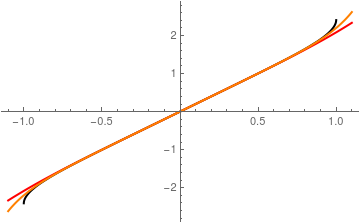Surprisingly, the graph of the function $$f(x) = \sin x + \arcsin x$$ looks like a straight line with slope of 2 in a neighborhood of the origin. It is hard to determine how good these approximations are from the graph, so we restrict ourselves with the interval [-0.5, 0.5] and estimate derivatives of the given function on this interval.
list = Table[D[f[x], {x, i}], {i, 5}]
Do[Print[FindMaximum[{list[[k]]/k!, -0.5 <= x <= 0.5}, x]], {k, 1, 5}]
{2.03228,{x->0.5}}
{0.145187,{x->0.5}}
{0.366936,{x->0.5}}
{0.61871,{x->0.5}}
{0.874051,{x->0.5}}
To estimate the error of approximation by polynomial $$s_5 (x) = 2x + \frac{x^5}{12}$$ on the interval [-0.5, 0.5], we need to evaluate the sixth derivative on this interval:
D[f[x], {x, 6}]/6! /. x -> 0.5
1.29184
Multiplying this number by 0.56, we obtain the final estimate:
%*0.5^6
0.020185
So the error is about 0.02 on this interval.    ▣

Example: We start with basic polynomial approximation of well-known sine function. First, we calculate its 10 and 20-th term polynomial approximations

s10[x_] = Normal[Series[Sin[x], {x, 0, 10}]]]
s20[x_] = Normal[Series[Sin[x], {x, 0, 20}]]
Then we plot these approximations together with the true sine function:
Plot[{Sin[x], s10[x], s20[x]}, {x, -10, 10}, PlotStyle -> {{Thick, Black}, {Thick, Red}, {Thick, Orange}}]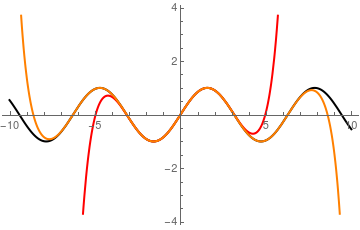So we see that more terms are used in Taylor's polynomial, the better approximation.

Now consider the 100th Maclaurin polynomial for sine function

poly = Normal[Series[Sin[x], {x, 0, 100}]];
N[Part[poly, {1, 2, 3, 5, -2, -1}]]
x - 0.166667 x^3 + 0.00833333 x^5 + 2.75573*10^-6 x^9 + 1.03958*10^-152 x^97 - 1.07151*10^-156 x^99
If we try to plot it, the result appears to break down past 35. After all, this is a polynomial of degree 100 and so it cannot have the more than 100 roots as indicated by the plot
Plot[poly, {x, 0, 100}, PlotRange -> {-2, 2}, PlotStyle -> {Thickness[0.0016], Black}]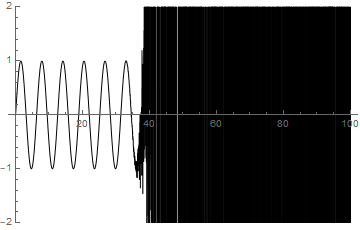To see why it is so difficult to get an accurate value, consider x = 50. The polynomial's value there is a rational number and we can compute it.
poly /. x -> 50  // Short
<<1>>
To get the numerical value, we type in
N[poly /. x -> 50]
-0.262375
But when we simply insert the approximate real number 50.0 into the polynomial, as Plot will do, we get a number that is more than a million times too large; this occurs because of the roundoff error in forming the sum using machine precision only
poly /. x -> 50.0
-100984.
Just to be clear, this sort of thing happens when small numbers mix with large ones in a machine precision environment. With no decimal point, the following would pose no problem. But the use of machine precision, caused by the decimal point, causes classic subtraction error. Now, we can increase the precision.
Precision[%]
76.3063
So one approach to getting an accurate plot is to use 100 digits of working precision.
Plot[poly, {x, 0, 100}, WorkingPrecision -> 100, PlotRange -> {-2.2, 1.2}]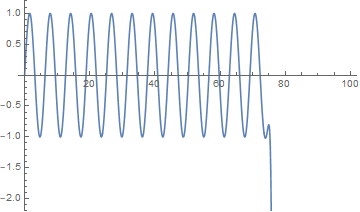This is not an ideal solution, because the user must know that 80 digits are enough, while 20 digits are not. It would be nice if one could get accurate answers in numerically unstable situations without having to understand anything about the exact nature of the instability. In fact, this is possible. To do this, we use Mathematica's adaptive precision:
PrecisePlot[f_, {x_, a_, b_}, opts___] := Module[{g, pg}, pg = PrecisionGoal  /. {opts} /. Options[PrecisePlot];
SetAttributes[g, NumericFunction];
g[z_?InexactNumberQ] := Evaluate[f /. x -> z];
Plot[N[g[SetPrecision[y, Infinity]], pg], {y, a, b}, Evaluate[Sequence @@ FilterRules[{opts}, Options[Plot]]]]];
PrecisePlot[poly, {x, 0, 80}, PlotRange -> {-2.4, 1.2}, MaxRecursion -> 3]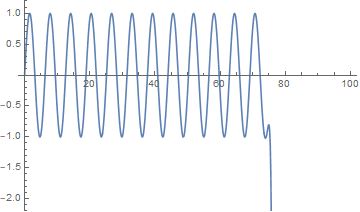Let us estimate the quality of Maclaurin approximation for sine function using the Lagrange reminder:
$R_{2n} (x) = \frac{f^{(2n)} (\xi )}{(2n)!} \, x^{2n} ,$
where ξ is some point from the interval |x| ≤ 𝑎. Using Stirling approximation for factorial $$n! \sim n^n \sqrt{2\pi\,n}\, e^{-n} ,$$ we estimate the remainder:
$\left\vert R_{2n} (x) \right\vert \le \frac{1}{(2n)!} \, a^{2n} \le \frac{a^{2n} e^{-2n}}{(2n)^{2n} \sqrt{2\pi\,2n}} \le \frac{a^{2n} e^{2n}}{(2n)^{2n}} = \left( \frac{e\,a}{2n} \right)^{2n} .$
To make this reminder less than ε for fixed n, we need to choose 𝑎 in accordance
$\frac{e\,a}{2n} \le \epsilon^{1/(2n)} \qquad \Longrightarrow \qquad a \le \frac{2n}{e} \,\epsilon^{1/(2n)} .$
In particular, if we want the Taylor's series
$T_9 (x) = x - \frac{x^3}{6} + \frac{x^5}{5!} - \frac{x^7}{7!} + \frac{x^9}{9!}$
to approximate the sine function on the interval |x| ≤ 𝑎 with an error of 10-6, we need to pick up the value of 𝑎 so that
$a \le \frac{10}{e} \,10^{0.6} \approx 0.924071 .$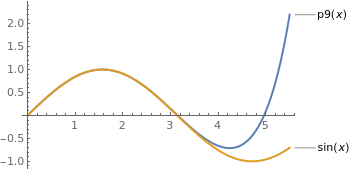Polynomial approximation of sinx. 10*Exp[-1]*10^(-0.6) p9[x_] = x - x^3/3! + x^5 /5! - x^7 /7! + x^9 /9! Plot[{p9[x], Sin[x]}, {x, 0, 5.5}, PlotStyle -> Thick, PlotLabels -> Automatic]
However, if we want 10% accuracy (which is enough for plotting), the value of 𝑎 should be close to 3.    ▣

Power Series Solutions to Differential Equations

Can Taylor series be used for practical determinations of ODE solutions? Not really because in most cases we don't know apriori the radius of convergence of such series (which depends on estimates of higher derivatives for an unknown solution). This means that for proper Taylor's approximation one needs to perform additional analysis. Remember that a Taylor's series uses only infinitesimal information of its sum-function, so we expect good approximation only in a small neighborhood of the center. Nevertheless, power series method becomes essential for numerical calculations for small domains close to the center of expansion. For example, the spline method usually uses cubic approximations locally. Fortunately, Mathematica has a dedicated command AsymptoticDSolveValue for determination of a power series approximation for a solution.

A differential equation establishes a relation between derivatives of unknown function and the function itself. When power series method is applied for solving differential equations, the main issue is to recover a relation between coefficients of the sum-function according to the given differential equation. Since the derivative of the solution represented by a power series

$f'(x) = \sum_{n\ge 1} n\,c_n \left( x - x_0 \right)^{n-1} = \sum_{n\ge 0} c_{n+1} \left( n+1 \right) \left( x - x_0 \right)^n$
makes a shift in its coefficients, the required relation between its coefficients becomes a recurrence or difference equation. This is the main reason why we discuss recurrences in the first part of this chapter. It should be noted that this topic is important in other branches of science, including numerical analysis.

The hunting license for finding solutions of differential equations in the form of power series gives the following famous theorem credited to Augustin Cauchy (1842) and Sophie Kovalevskaya (1875). We present its simple version; however, the reader can find its numerous extensions elsewhere. The Cauchy--Kovalevskaya theorem does not provide explicitly the radius of convergence for series solution to the initial value problem. Therefore, it has only theoretical meaning and we need to find other resources for its identification.

Theorem Cauchy--Kovalevskaya: If f(x, y) can be developed by Taylor's theorem as a power series in x - x0 and y - y0, absolutely convergent when the absolute values of these elements do not exceed certain definite amounts, then there exists a solution to the differential equation $$y' = f(x,y)$$ in the form of convergent infinite series $$y = y(x) = y_0 + \sum_{n\ge 1} c_n \left( x- x_0 \right)^n ,$$ which satisfies the initial condition y(x0) = y0. This solution is unique in the class of real analytic functions.

The above existence theorem gives a sufficient condition for a unique solution, and, moreover, it suggests a possible form for that solution. In general, failure to satisfy the conditions of the above theorem does not prevent the existence of a holomorphic solution. The German mathematician Karl Theodor Wilhelm Weierstrass (1815--1897) proved in 1885 the following theorem.

Weierstrass approximation theorem: Every continuous function defined on a closed interval [𝑎, b] can be uniformly approximated as closely as desired by a polynomial function.

Mathematica has a dedicated command to find power series expansion of the solution to the initial value problem: AsymptoticDSolveValue.

Example: Consider the (separable) differential equation

$\frac{{\text d}y}{{\text d} x} = \frac{1 -2\,x\,\sqrt{3} + 3x^2}{3\,y^2}$
that has the general solution $$y = \sqrt{c + x -x^2 \sqrt{3} + x^3} .$$ Here the slope function tends to infinity when x = x0, y = 0. In order to get y = 0 when x = x0, we must set c = -x0 + x² 3½ - -x0³. This yields the single solution $$y = \sqrt{x - x_0 + x^3 - x_0^3 - \left( x - x_0 \right)^2 \sqrt{3}} ,$$ which is not developed by Taylor's series in powers of x - x0 except when $$x_0 = 1/\sqrt{3}$$ because in this case the problem has a linear solution $$y = x - 1/\sqrt{3} .$$
Simplify[(1 - 2*x*Sqrt + 3*x^2)/(x - 1/Sqrt)^2/3]
1
■

A power series $$\phi (x) = \sum_{n\ge 0} c_n \left( x - x_0 \right)^n$$ converges (absolutely) within a symmetric interval $$\left\vert x - x_0 \right\vert < R ,$$ and diverges outside. If R is a positive number, then we say that the power series converges; if R = 0, the series diverges everywhere except the center x0. The number R is called the radius of convergence. If the solution to a differential equation is represented by a power series, its radius of convergence not only limits its validity interval, but also gives a qualitative description of the sum-function. The radius of convergence is the distance to the nearest singular point, which may belong to the boundary of the validity interval or may not when the sum has either a complex singularity or a branch point. Generally speaking, the radius of convergence can not be determined from the validity interval. Singular points also affect numerical algorithms used to approximate solutions.

Over the past two hundred years, some equations are so frequent in the physical applications that their series solutions have led to the introduction of new functions (Bessel, hypergeometric, etc.). The solutions (in the series form) to these equations have been determined and their properties have been intensively studied. The corresponding branch of mathematics is called the theory of special functions, which is devoted to the study and applications of functions not expressible through elementary functions. The majority of these special functions are solutions of differential equations with singular points where the coefficients are undefined. These equations were studied for the first time by Briot and Bouquet in 19-th century. Below are some examples of singular differential equations.

Example: Consider the differential equation $$y' = y/x ,$$ where the slope function has a singular point at the origin. Nevertheless, this equation has the polynomial general solution y = cx, with an arbitrary constant c, which is undefined at the origin. Therefore, it is impossible to assign a value at this point. To knock out a single solution, we have to impose an initial condition at some point but not the origin.

A similar differential equation $$y' + y/x^2 =0$$ has the general solution $$y = c\,e^{1/x}$$ that is undefined at the origin.

The differential equation $$y' = 1 + y/x$$ has the general solution $$y = x\,\ln x + cx .$$ Here y takes the value 0 when x = 0. But it is impossible to express the solution in the form of a Taylor series in powers of x. In this case, we have an indefinite number of solutions for the one initial value 0, and no solution for any other initial value of y when x = 0.

■

As the above example shows, differential equations with initial conditions specified at the singular point may lead to serious circumstances---even of impossibility---to define a solution at these points. Even when initial an condition can be specified at the singular point, the corresponding initial value problem for such equation with the initial condition at the singular point may have multiple solutions or may not have a holomorphic solution. Therefore, at the end of this chapter, we will discuss initial value problems for a certain class of linear singular equation, called Fuchsian equations.

Example: We demonstrate Mathermatica's capability to determine a power series expansion of the solution to the first order Riccati equation:

$y' = x^2 - y^2 , \qquad y(0) = 1.$
When you try to find its solution with Mathematica
DSolve[{y'[x] + y[x]^2 == x^2, y == 1}, y[x], x]
you will realize that there is no support from the CAS. The basic Mathermatica command AsymptoticDSolveValue allows one to find a power series expansion of the solution without actually solve the corresponding initial value problem.
AsymptoticDSolveValue[{y'[x] + y[x]^2 == x^2, y == 1}, y[x], {x, 0, 10}]
1 - x + x^2 - (2 x^3)/3 + (5 x^4)/6 - (4 x^5)/5 + (23 x^6)/30 - ( 236 x^7)/315 + (201 x^8)/280 - (218 x^9)/315 + (2803 x^10)/4200
So we know the first ten terms in its Maclaurin series expansion.

To develop a truncated power series solution, we use another approach. First, we define the differential equation and the initial condition:

ode = y'[x] + y[x]^2 == x^2; initconds = {y == 1};
Then we create a differential operator corresponding to the given differential equation:
odeOperator = D[#, {x, 1}] + #^2 - x^2 &;
Now set up our Taylor series as a symbolic expansion using derivatives of y(x) evaluated at the origin.
xx = Series[y[x], {x, 0, 6}]
y + y' + y''/2 + y'''/6 +
Next apply the differential operator and add the initial conditions. Then find a solution that makes all powers of x vanish.
soln = SolveAlways[Join[{odeOperator[xx] == 0}, initconds], x]
Let's look at the Taylor polynomial.
truncatedSol = Normal[xx /. soln[]]
1 - x + x^2 - (2 x^3)/3 + (5 x^4)/6 - (4 x^5)/5
Now we plot the truncated power series solution in red and its solution in blue:
approxSol = NDSolve[Join[{ode}, initconds], y[x], {x, 0, 3}];
Plot[{truncatedSol, y[x] /. approxSol[]}, {x, 0, 2}, PlotStyle -> {{Thick, Red}, {Thick, Blue}}]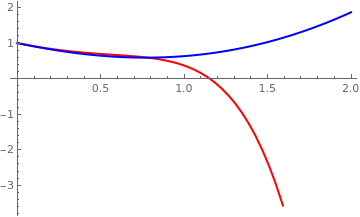We can find its series expansion in another way.

y = 1 + Sum[a[i] x^i , {i, 5}] + O[x]^6
1 + a x + a x^2 + a x^3 + a x^4 + a x^5 + SeriesData[ x, 0, {}, 0, 6, 1]
D[y, x] + y^2 == x^2
LogicalExpand[%];
Solve[%]
{{a -> -1, a -> 1, a -> -(2/3), a -> 5/6, a -> -(4/5)}}
▣
Also, the linear differential equations can be solved in terms of power series using the command DifferentialRoot:
lde = {y''[x] - x*x*y'[x] + Cos[x] == 0, y' == 1, y == 0};
Series[DifferentialRoot[Function @@ {{y, x}, lde}][x], {x, 0, 15}]
x - x^2/2 + x^4/8 - x^5/20
We can write the script to determine the series expansion of a second order differential equation.
Clear[seriesDSolve];
seriesDSolve[ode_, y_, iter : {x_, x0_, n_}, ics_: {}] :=
Module[{dorder, coeff}, dorder = Max[0, Cases[ode, Derivative[m_][y][x] :> m, Infinity]];
(coeff = Fold[Flatten[{#1, Solve[#2 == 0 /. #1,
Union@Cases[#2 /. #1, Derivative[m_][y][x0] /; m >= dorder, Infinity]]}] &, ics,
Table[SeriesCoefficient[ ode /. Equal -> Subtract, {x, x0, k}], {k, 0, Max[n - dorder, 0]}]];
Series[y[x], iter] /. coeff) /; dorder > 0]
and the apply to the initial value problem:
seriesDSolve[ y''[x] + y'[x] == Sin[3 y[x]], y, {x, 0, 5}, {y -> 1, y' -> 0}]
We can apply the standard Mathematica command
ode = x''[t] - t*t*x'[t] + Cos[t] == 0;
initconds = {x' == 1, x == 0};
AsymptoticDSolveValue[{ode, initconds}, x[t], {t, 0, 8}]
We create a differentail operator to generate this ODE:
odeOperator = D[#, {t, 2}] - t*t*D[#, t] + Cos[t] &;
Now set up our Taylor series as a symbolic expansion using derivatives of x evaluated at the origin. I use an order of 8 but that is something one would probably make as an argument to a function, if automating all this.
xx = Series[x[t], {t, 0, 8}];
Next apply the differential operator and add the initial conditions. Then find a solution that makes all powers of `t vanish.
soln = SolveAlways[Join[{odeOperator[xx] == 0}, initconds], t];
Let's look at the Taylor polynomial:
truncatedSol = Normal[xx /. soln[]]
To assess how accurate it might be I will compare with NDSolve
approxSol = NDSolve[Join[{ode}, initconds], x[t], {t, 0, 4}];
Plot[{Callout[truncatedSol, "polynomial"], Callout[x[t] /. approxSol[], "true solution"]}, {t, 0, 3}, PlotTheme -> "Web"]

Radius of convergence for the series solution

Suppose we are given a linear second order differential equation
\begin{equation} \label{EqTaylor.5} a_2 (x)\,y'' + a_1 (x) \,y' + a_0 (x)\,y = f(x) , \end{equation}
where 𝑎0, 𝑎1, 𝑎2, and f(x) are holomorphic functions centered at the point x = x0. Then Eq.\eqref{EqTaylor.5} has a series solution
\begin{equation} \label{EqTaylor.6} y(x) = \sum_{n\ge 0} c_n \left( x - x_0 \right)^n \end{equation}
that converges in the circle |x - x0| < ρ of radius ρ that is the distance to the nearest singularity of each of the functions 𝑎0, 𝑎1, 𝑎2, and f(x).

Example: Consider the differential equation

$\left( 4 + x^2 \right) y'' + 3x\,y' -x^2 y = 0 .$
If we were given the initial conditions at the origin, we expect the solution of the corresponding initial value problem to be represented by power series:
$y(x) = \sum_{n\ge 0} c_n x^n .$
Roots of 4 + x² = 0 are x = ±j (where j is the unit vector in positive vertical direction on the complex plane ℂ), so we expect the radius of convergence of Taylor's series for (4+x-1) to be 2 because
$\frac{1}{4+x^2} = \frac{1}{4} \,\frac{1}{1+x^2 /4} = \frac{1}{4} \left( 1 - \frac{x^2}{4} + \frac{x^4}{2^4} - \cdots \right) ,$
and using the ratio test, we get
$\lim_{n\to\infty} \left\vert \frac{a_{n+2}}{a_n} \right\vert = \lim_{n\to\infty} \left\vert \frac{x{2n+2}/2^{2n+2}}{x^{2n}/2^{2n}} \right\vert = \left\vert \frac{x^2}{2^2} \right\vert < 1 \quad\Longrightarrow \quad |x| < 2 .$

If the initial conditions were given at the point x = 1, then a power series expansion of the form $$\displaystyle \sum_{n\ge 0} c_n \left( x-1 \right)^n$$ is required. The distance from the point x = 1 till singular point x = 2j is $$\displaystyle \sqrt{5} .$$ So the radius of convergence of the series $$\displaystyle \sum_{n\ge 0} c_n \left( x-1 \right)^n$$ is 51/2.    ■

1. Allame, M. and Azad, N., Solution of Third Order Nonlinear Equation by Taylor Series Expansion, World Applied Sciences Journal, 14 (1): 59-62, 2011.
2. Bervillier, C., Status of the differential transformation method, Applied Mathematics and Computation, 2012, Volume 218, Issue 20, 15 June 2012, Pages 10158-10170; https://doi.org/10.1016/j.amc.2012.03.094
3. Dettman, J.W., Power Series Solutions of Ordinary Differential Equations, The American Mathematical Monthly, 1967, Vol. 74, No. 3, pp. 428--430.
4. Driscoll, T.A., Hale, N., and Trefethen, L.N. editors, Chebfun Guide, Pafnuty Publications, Oxford, 2014.
5. Gray, J.J., Fuchs and the theory of differential equations, Bulletin of the American Mathematical Society, 1984, Vol. 10, No. 1, pp. 1--26.
6. Grigorieva, E., Methods of Solving Sequence and Series Problems, Birkhäuser; 1st ed. 2016.
7. Hirschman, I.I., Infinite Series, Dover Publications, 2014.
8. Knopp, K., Theory and Application of Infinite Series, Dover Publications, 1990.
9. von Kowalevsky, Sophie (1875), Zur Theorie der partiellen Differentialgleichung, Journal für die reine und angewandte Mathematik, Vol. 80, pp. 1–32. (German spelling of her surname used at that time.)
10. Kudryashov, N.A., On one of methods for finding exact solutions of nonlinear differential equations,
11. Liao, S. and Tan, Y., A general approach to obtain series solutions of nonlinear differential equations, Studies in Applied Mathematics, 119(4, pp. 297--354.
12. Piaggio, H.T., An Elementary Treatise on Differential Equations and their Applications, Revised edition, 1952, London, G.Bell & Sons Ltd.
13. Robin, W., Frobenius series solution of Fuchs second-order ordinary differential equations via complex integration, International Mathematical Forum, 2014, Vol. 9, 2014, no. 20, 953--965; http://dx.doi.or g/10.12988/imf.2014.4491
14. Simmons, G.F., Differential equations with applications and historical notes, 1972, McGraw-Hill, New York. QA372.S49
15. Trefethen, L.N., Six myths of polynomial interpolation and quadrature, Mathematics Today 47 (2011), 184-188.